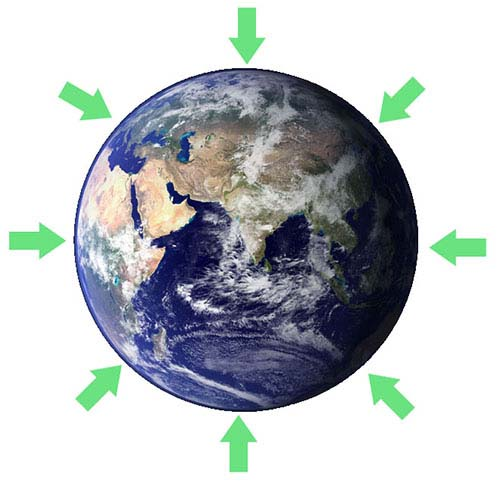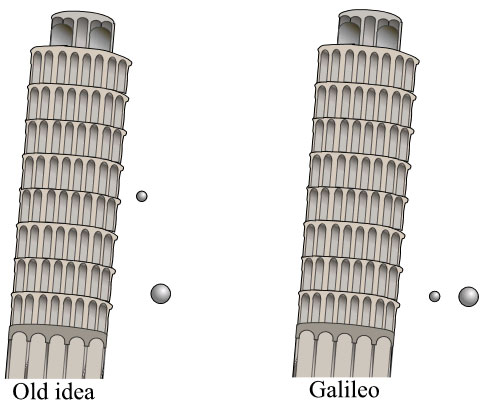Have you ever tried to jump?

Probably, yes! And you know that when we jump from the ground, we just fall back after few seconds.Why? And How?

These are the questions that, almost every human had thought in his childhood. So, the simple answer to all these questions is “gravity”.

So, let’s learn something about gravity and the interesting history about it.

What is Gravity?

Gravity is the force that attracts us and other objects towards each other; much like a magnet, but not as a magnet. Then, why things in your room are not attracted to each other? It is because gravity depends on the mass; Massive objects have more gravity.

Gravitational force is so weak that the object must be very massive to have sufficiently strong gravity to attract other objects. Consider our earth, it so massive that its gravity pulls us towards the ground. When we jump, earth’s gravity pulls us and we fall back on the ground.

Importance of Gravity

What would happen, if gravity suddenly disappears? Take some time and think about it.You will be amazed to find out that, the current appearance of our earth, planets, and stars depends on the gravity. Without gravity, there will be no earth’s pull to attract you back to the ground when jumping. Even the planets and stars would not exist without gravity. Because planets and stars have been formed from the accumulation of cosmic dust and gas that was only possible due to gravity.

Here are some of the effects of gravity on our everyday life

• We can stand, jump, walk, and run on the ground due to gravity. If the earth doesn’t pull you back while jumping, then you would never come back to the ground, and you may reach space in a single jump! Wow!
• On the earth, gravity keeps the air around us as a blanket, and it prevents air from escaping into space.
• Tides in the oceans are caused by the gravitational pull of moon and sun.

Universal Law of Gravitation

It is just a simple law that was proposed by a famous scientist Sir Isaac Newton. This law gives us the relation between the gravitational force, the mass of objects, and the distance between objects.

Here are two simple points that explain the law:

• The gravitational force between massive objects is more as compared to light objects.
• The gravitational force between two objects decreases exponentially as they go away from themselves.

Here is the mathematical expression of the universal law of gravitation:

F = G*M1*M2/r^2

G = Gravitational constant, whose value is equal to
M1 = mass of the first object
M2 = mass of the second object
r = distance between two objects

By knowing gravity, you can calculate when a ball will touch the ground after being released into the air. You can measure and graph the path of a projectile. You can calculate how much propellant is required to push a rocket into space, and many more.

Do Heavy and Light Objects Fall at the Same Time?The simple answer is, yes. But let’s understand, why it happens.

Gravity at the surface of the earth is 9.8 m/s, which means an object falling towards earth gains 9.8 m/s speed in every second. If a ball is dropped from a building it will have 9.8 m/s speed at the end of the 1st second, 19.6 m/s at end of the 2nd second, and 29.4 at the end of the 3rd second and so on. Every object is accelerated at the same rate towards earth, whether it is a paper clip or a stone. If two objects are dropped from a height, they both will hit the ground at the same time.

But, wait a minute!

You may be thinking, why a paper falls slower than a paper clip when both are dropped from the same height? It is because of the air resistance. The paper clip has lower surface area than the paper, which results in higher air resistance for the paper. But, if you drop paper and paper clip into a vacuum chamber both will hit the bottom at the same time. It is because vacuum chamber does not have air, so there will be no air friction to slow any object.

Difference between Mass and WeightIn everyday life, you may have used the term mass and weight interchangeably. But in physics, mass and weight are two different quantities.

The mass of an object is the quantity of matter in it; usually measured in kilograms. But, weight is the force of an object that it is putting on a platform due to gravity.

For example, if you hold a brick in your hand you will feel a downward force. If you somehow take that brick to the moon, you will notice much less downward force. Because the moon has less gravitation than the earth. In both cases, the amount of the matter in the brick will be same on the earth and the moon.

Difference between Gravity and Gravitation

There is a difference between gravity and gravitation. Gravitation is the force of attraction between objects that have mass. But, gravity is the gravitational force between earth and other objects. It means that gravity is the special case of gravitation.

Gravitational force exists since the formation of our universe. It is one of the four fundamental forces of nature. It is the force that attracts atoms together to form stars and planets. It keeps the moon in orbit around the earth and the earth around the sun.

Fun Facts

• Gravity keeps all the planets in the orbit of the sun, and it keeps our moon in orbit of the earth.
• If any object goes upward with a velocity of 11 km/s, it will escape the gravitational pull of the earth. This velocity is called the escape velocity, and it is different for different planets and moon.
• The gravity of our sun is about 274 m/s^2. Anything weighing 1 kg on the earth will weigh about 28 kg (If it was possible!).

A Brief History

Let’s learn something about the history of gravity, and find out about the people who contributed to explaining the gravity.

Who first pointed to the presence of gravity? And when? It is still unknown. But you may consider that early human beings had probably thought about it. Because, it is the force whose effects we can clearly see, like falling back to the ground after jumping.

• The first person who gave the hypothesis about gravity was, Aristotle. He hypothesized that “heavier objects are accelerated more towards ground than lighter objects”. He was actually wrong because he didn’t know about air resistance which slows down the objects. But, he at least provided the ground for the great scientists to think and research about gravity.